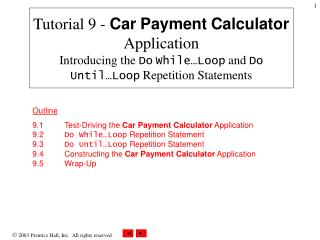DownloadDownload PresentationTutorial 9 - Car Payment Calculator Application Introducing the Do While…Loop and Do Until…Loop Repetition Stateme

# Tutorial 9 - Car Payment Calculator Application Introducing the Do While…Loop and Do Until…Loop Repetition Stateme

Télécharger la présentation## Tutorial 9 - Car Payment Calculator Application Introducing the Do While…Loop and Do Until…Loop Repetition Stateme

- - - - - - - - - - - - - - - - - - - - - - - - - - - E N D - - - - - - - - - - - - - - - - - - - - - - - - - - -
##### Presentation Transcript

1. Tutorial 9 - CarPaymentCalculator ApplicationIntroducing the DoWhile…Loop and DoUntil…Loop Repetition Statements Outline 9.1 Test-Driving the Car Payment Calculator Application9.2 Do While…Loop Repetition Statement9.3 Do Until…Loop Repetition Statement9.4 Constructing the Car Payment Calculator Application9.5 Wrap-Up

2. Objective • In this tutorial, you will learn to: • Use the Do While…Loop and Do Until…Loop repetition statements to execute statements in a program repeatedly. • Use counter-controlled repetition. • Display information in ListBoxes.

3. 9.1 Test-Driving the Car Payment Calculator Application

4. ListBox control 9.1 Test-Driving the Car Payment Calculator Application Figure 9.1Car Payment Calculator application before data has been entered.

5. 9.1 Test-Driving the Car Payment Calculator Application Figure 9.2Car Payment Calculatorapplication after data has been entered. • Input data • Click CalculateButton

6. Results displayed in tabular format 9.1 Test-Driving the Car Payment Calculator Application Figure 9.3　Car Payment Calculator application displaying calculation results.

7. 9.2 Do While…Loop Repetition Statement • Loop-continuation condition • While loop-continuation condition remains true, loop statement executes its body repeatedly • When loop-continuation condition becomes false, loop terminates • Code example: – Dim intProduct As Integer = 3Do While intProduct <= 50 intProduct *= 3Loop

8. 9.2 Do While…Loop Repetition Statement Figure 9.4　Do While Loop repetition statement UML activity diagram.

9. 9.3 Do Until…Loop Repetition Statement • Loop-continuation condition • While loop-continuation condition remains false, loop statement executes its body repeatedly • When loop-continuation condition becomes true, loop terminates • Code example: – Dim intProduct As Integer = 3Do Until intProduct > 50 intProduct *= 3Loop

10. 9.3 Do Until…Loop Repetition Statement Figure 9.5　Do Until Loop repetition statement UML activity diagram

11. 9.4 Constructing the Car Payment Calculator Application

12. 9.4 Constructing the Car Payment Calculator Application

13. 9.4 Constructing the Car Payment Calculator Application Figure 9.7Car Payment Calculatorapplication’s Form in design mode.

14. ListBox’s control name displayed in design view 9.4 Constructing the Car Payment Calculator Application • ListBoxcontrol • Change the Name property Figure 9.8　ListBox added toCar Payment Calculatorapplication’s Form.

15. 9.4 Constructing the Car Payment Calculator Application • Adding code to the event handler • Clearing the ListBox • Call method Clear on property Items • Items property returns an object containing items in ListBox

16. 9.4 Constructing the Car Payment Calculator Application Figure 9.9　Clearing the contents of aListBox.

17. 9.4 Constructing the Car Payment Calculator Application • Adding a header to the ListBox • Call method Add • String-concatenation operator (&) • ControlChars.Tab constant

18. 9.4 Constructing the Car Payment Calculator Application Figure9.10Adding a header to aListBox.

19. Variables to store the length of the loan Variables to store userinput Variables to store calculation results 9.4 Constructing the Car Payment Calculator Application Figure9.11　Variables for theCar Payment Calculatorapplication. • Variable declarations

20. 9.4 Constructing the Car Payment Calculator Application Figure9.12　Retrieving input in theCarPayment Calculatorapplication. • Retrieving input • Use Valfunction • Divide interest rate by100

21. 9.4 Constructing the Car Payment Calculator Application • Calculating values used in the calculation • Subtract down payment from price • Monthly interest rate is interest divided by 12

22. 9.4 Constructing the Car Payment Calculator Application Figure9.13　Determining amount borrowed and monthly interest rate.

23. 9.4 Constructing the Car Payment Calculator Application • Setting the loop-continuation condition • Counter-controlled repetition • Uses a counter variable • Also called definite repetition

24. 9.4 Constructing the Car Payment Calculator Application Figure9.14　Loop-continuation condition.

25. 9.4 Constructing the Car Payment Calculator Application Figure9.15　Converting the loan duration from years to months. • Add the length of the loan in months

26. 9.4 Constructing the Car Payment Calculator Application • Calculate monthly payment amount • Use Pmt function • Returns a Double value • Type Decimal stores monetary values • Use the Convert.ToDecimal method

27. 9.4 Constructing the Car Payment Calculator Application Figure9.16　Pmt function returns monthly payment.

28. 9.4 Constructing the Car Payment Calculator Application • Displaying the result • Use method Add • Use method String.Format to display values in currency format

29. 9.4 Constructing the Car Payment Calculator Application Figure9.17　Displaying the number of months and the amount of each monthly payment.

30. 9.4 Constructing the Car Payment Calculator Application • Increment the counter • Add one to the counter • Will terminate the loop when value becomes 6

31. 9.4 Constructing the Car Payment Calculator Application Figure9.18　Incrementing the counter.

32. CarPayment-Calculator.vb(1 of 3)

33. CarPayment-Calculator.vb(2 of 3)

34. CarPayment-Calculator.vb(3 of 3)